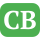CppBuzz.com

# C++ Interview Questions on Time & Space Complexity : (9) Questions

Question: 1

(A) O(log N)
(B) O(N/2)
(C) O(N)
(D) O(N^2)

Compiler
Level: Beginner Category: time_space_complexity
[Posted by: CppBuzz | Chicago, USA]

Question: 2

(A) Compilation error
(B) O(logN)
(C) O(N/2)
(D) O(N)

Compiler
Level: Practionar Category: time_space_complexity
[Posted by: CppBuzz | Chicago, USA]

Question: 3

(A) O(2N)
(B) O(N)
(C) O(N+N)
(D) O(N*N)

Compiler
Level: Practionar Category: time_space_complexity
[Posted by: CppBuzz | Chicago, USA]

Question: 4

(A) O(N+M)
(B) O(N*M)
(C) O(N)
(D) O(M)

Compiler
Level: Practionar Category: time_space_complexity
[Posted by: CppBuzz | Chicago, USA]

Question: 5

(A) O(N)
(B) O(Nlogn)
(C) O(N^2)
(D) O(N^3)

Compiler
Level: Practionar Category: time_space_complexity
[Posted by: CppBuzz | Chicago, USA]

Question: 6

(A) O(111*N)
(B) O(Nlogn)
(C) O(1)
(D) O(N)

Compiler
Level: Practionar Category: time_space_complexity
[Posted by: CppBuzz | Chicago, USA]

Question: 7

(A) O(N)
(B) O(logN)
(C) O(sqrt(N))
(D) O(∞)

Compiler
Level: Practionar Category: time_space_complexity
[Posted by: CppBuzz | Chicago, USA]

Question: 8

(A) O(LogN)
(B) O(1)
(C) O(N)
(D) O(N longN)

Compiler
Level: Practionar Category: time_space_complexity
[Posted by: CppBuzz | Chicago, USA]

Question: 9

(A) O(LogN) , O(N)
(B) O(N), O(N)
(C) O(N), O(1)
(D) O(N), O(0)

Compiler
Level: Practionar Category: time_space_complexity
[Posted by: CppBuzz | Chicago, USA]

### Browse C++ Categories

Loops Conditional Functions Operators Structure Enum Data Types Inheritance Pointers Classes & Objects Vtable Type Casting New & Delete Namespaces Virtual Functions Abstract Class STL Singleton Class Exceptions C++ 11 C++ 17 Time & Space Complexity others

Switch to C Questions